# Linear Programming in Python: Optimization Techniques ExplainedLinear programming is a technique that employs mathematical principles to identify the optimal solution for a problem that has multiple restrictions or limitations. It helps organizations efficiently allocate resources, minimize costs, and maximize profits by finding the optimal solution to linear constraints.

This article will extensively guide using Python to solve linear programming problems. We’ll cover formulating a problem, using popular Python libraries to find the optimal solution, and advanced topics in linear programming. This article is a valuable resource for students learning about optimization and professionals looking to apply linear programming in their work.

## Formulating A Linear Programming Problem

To formulate a linear programming problem, we need to follow several steps:

• Identify the decision variables: These are the variables that represent the quantities that we can change to achieve the optimal solution.
• Define the optimization function: This is the function we aim to increase or decrease, and it should be a linear combination of the variables we are trying to optimize.
• Define the limitations: The optimized variables must meet requirements to consider viable solutions.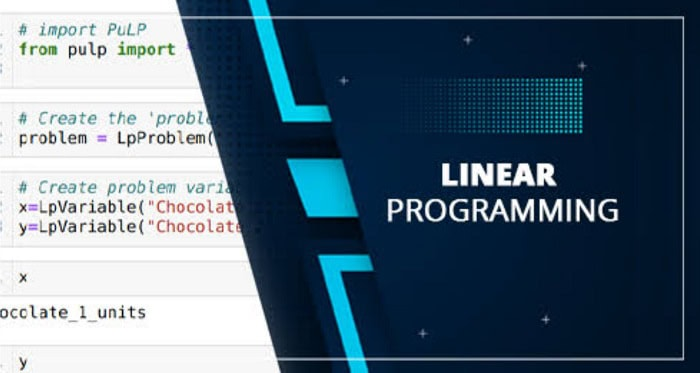By adhering to these procedures, we can create a linear programming problem that we can solve through optimization techniques.

## Solving A Linear Programming Problem In Python

Several Python libraries can solve linear programming problems. One such library is PuLP, a popular choice for linear programming in Python. PuLP allows you to formulate and solve problems using a simple syntax quickly. Here is an example of how to use PuLP to solve a linear programming problem: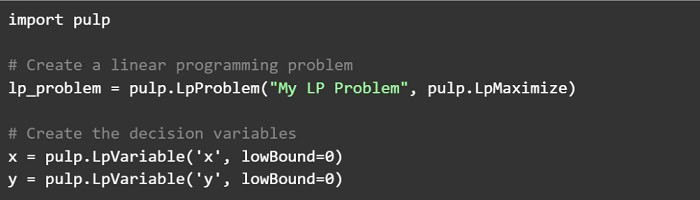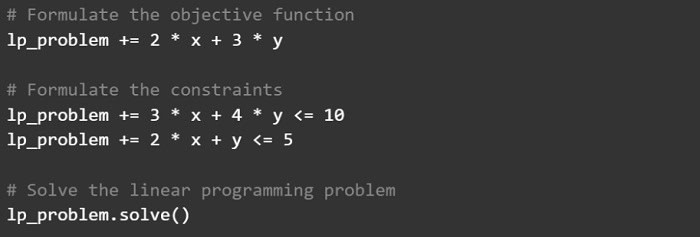In this example, we have defined two decision variables, x, and y, with lower bounds of 0. We have also formulated the objective function 2 * x + 3 * y to maximize and the constraints 3 * x + 4 * y <= 10 and 2 * x + y <= 5. Finally, we can use the solve() method to find the optimal solution to the linear programming problem. Then use the value() function to calculate the optimal x and y values.

Many other libraries in Python can be used to solve linear programming problems. However, PuLP is a simple and easy-to-use library well-suited for beginners.

## Advanced Topics in Linear Programming

Linear programming has various advanced topics that can improve our understanding of the technique and its practical applications.

• Sensitivity analysis shows how a problem’s optimal solution changes as its parameters vary within feasible ranges.
• Duality in linear programming refers to the relationship between the original problem (the primal problem) and a related problem that can also be solved (the dual problem).
• Integer programming optimizes over integer variables and is helpful for problems with discrete variables.

These concepts can be implemented in Python using libraries like PuLP. For Example-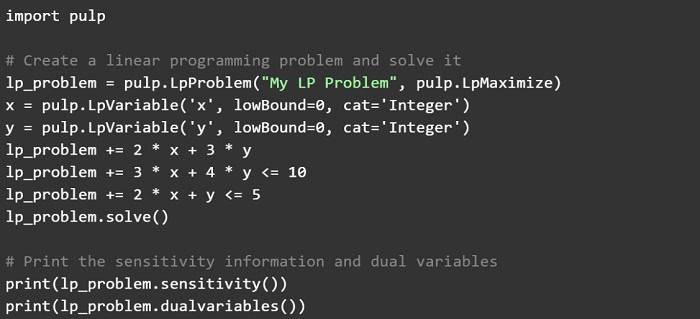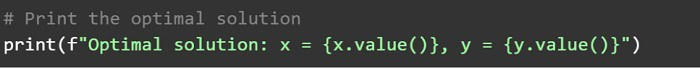In this code snippet, we have defined two integer variables, x, and y, and formulated a linear programming problem to maximize the objective function 2 * x + 3 * y. We have also solved the problem and printed the sensitivity information, dual variables, and optimal solution. These advanced topics can provide valuable insights into the structure and behavior of linear programming problems.

## FAQs

### How Do You Formulate A Linear Programming Problem In Python?

To formulate a linear programming problem in Python, you must define the objective function, the decision variables, and the constraints. The objective function is the function you want to optimize, while the decision variables are the variables you can control. The constraints are the conditions that limit the values that the decision variables can take.

### What Common Optimization Problems Can Be Solved Using Linear Programming In Python?

Some common optimization problems that can be solved using linear programming in Python include the transportation problem, the assignment problem, the production planning problem, and the diet problem.

### How Do You Solve A Linear Programming Problem In Python?

To solve a linear programming problem in Python, you can use the simplex algorithm, a standard linear programming algorithm. You can also use Python libraries such as PuLP, CVXOPT, and SciPy to solve linear programming problems.

### How Do You Visualize The Results Of A Linear Programming Problem In Python?

You can visualize the results of a linear programming problem in Python using Matplotlib or other Python visualization libraries. You can create graphs and charts to show the optimal solution and how the decision variables change as the constraints are varied.

### How Do You Handle Multiple Objective Functions In A Linear Programming Problem In Python?

You can use the weighted sum method or the epsilon-constraint method to handle multiple objective functions in a linear programming problem in Python. These methods involve combining the different objective functions into a single objective function that can be optimized using linear programming.

### What Are Some Limitations Of Linear Programming In Python?

Some limitations of linear programming in Python include the assumption of linearity, the requirement of continuous variables, and the difficulty of handling large-scale problems. Nonlinear programming and other optimization techniques may be required for more complex problems.

## Conclusion

This article presented an in-depth guide to linear programming using Python. We covered how to formulate and solve a linear programming problem using Python libraries and explored advanced topics such as sensitivity analysis, duality, and integer programming. Some key points from the article include:

• To find a linear objective function’s maximum or minimum value subject to linear constraints, we can use the optimization technique of Linear programming.
• Several Python libraries are available for addressing linear programming issues, including PuLP and Scipy. Optimize, and cvxpy.
• Advanced topics in linear programming include sensitivity analysis, duality, and integer programming.